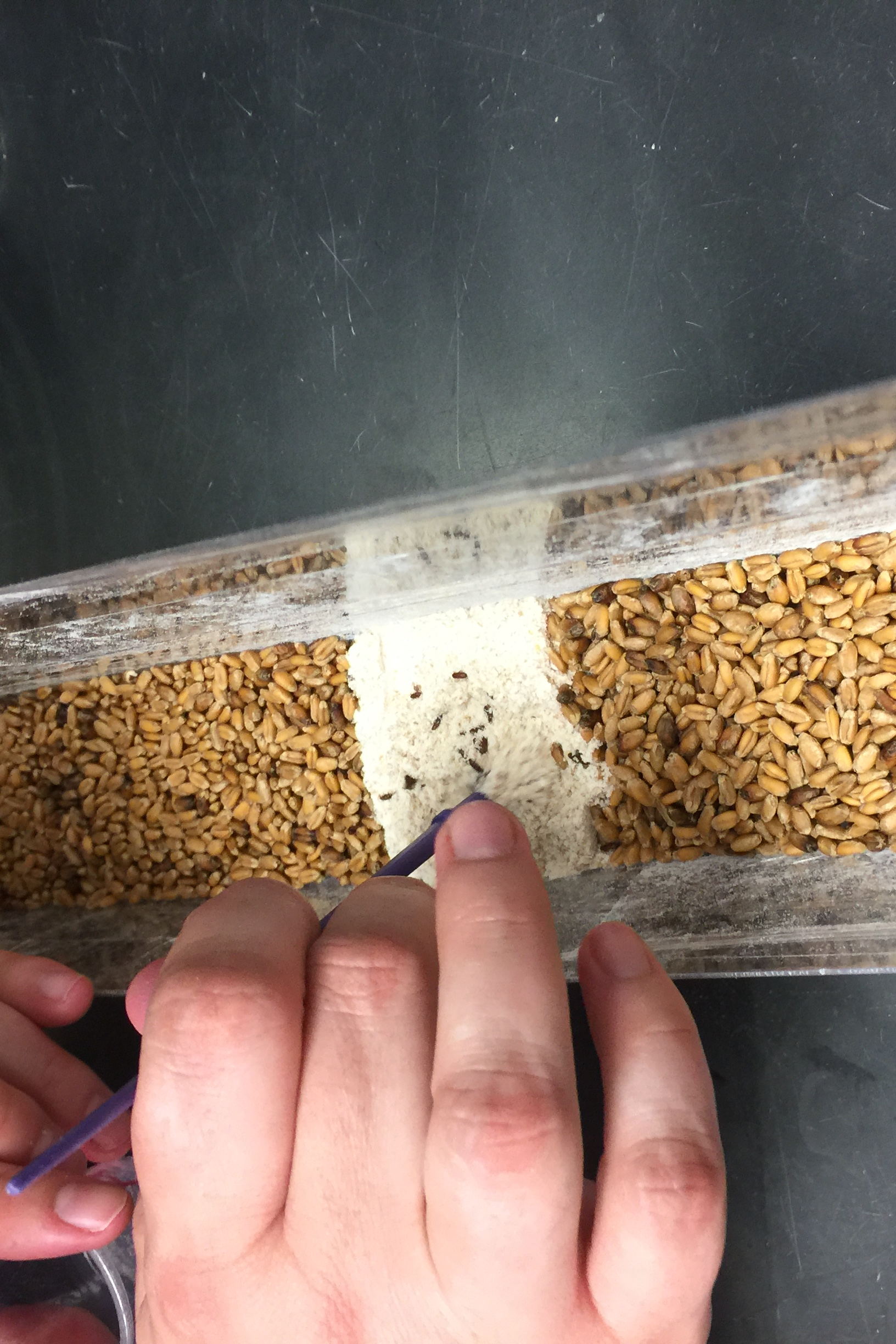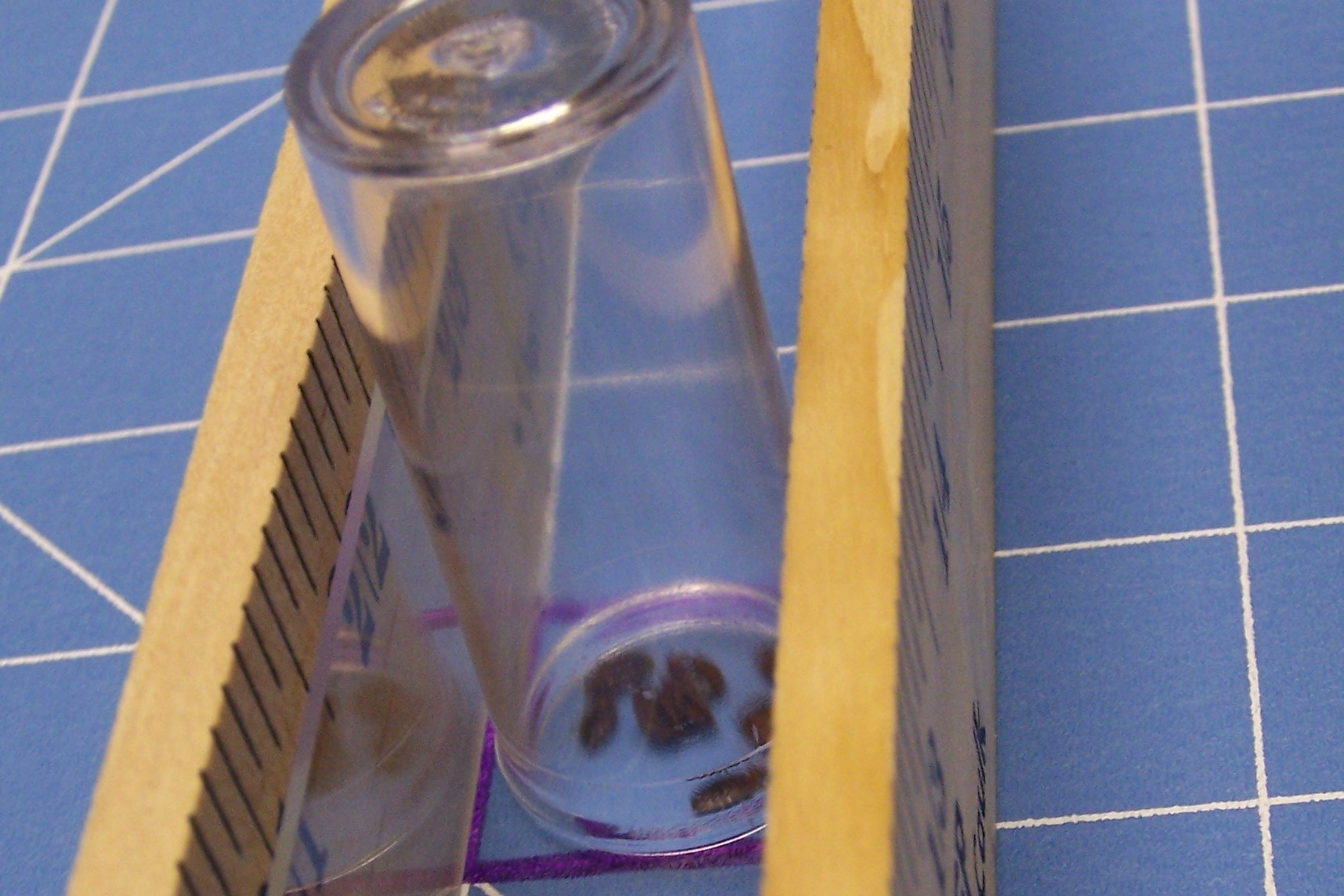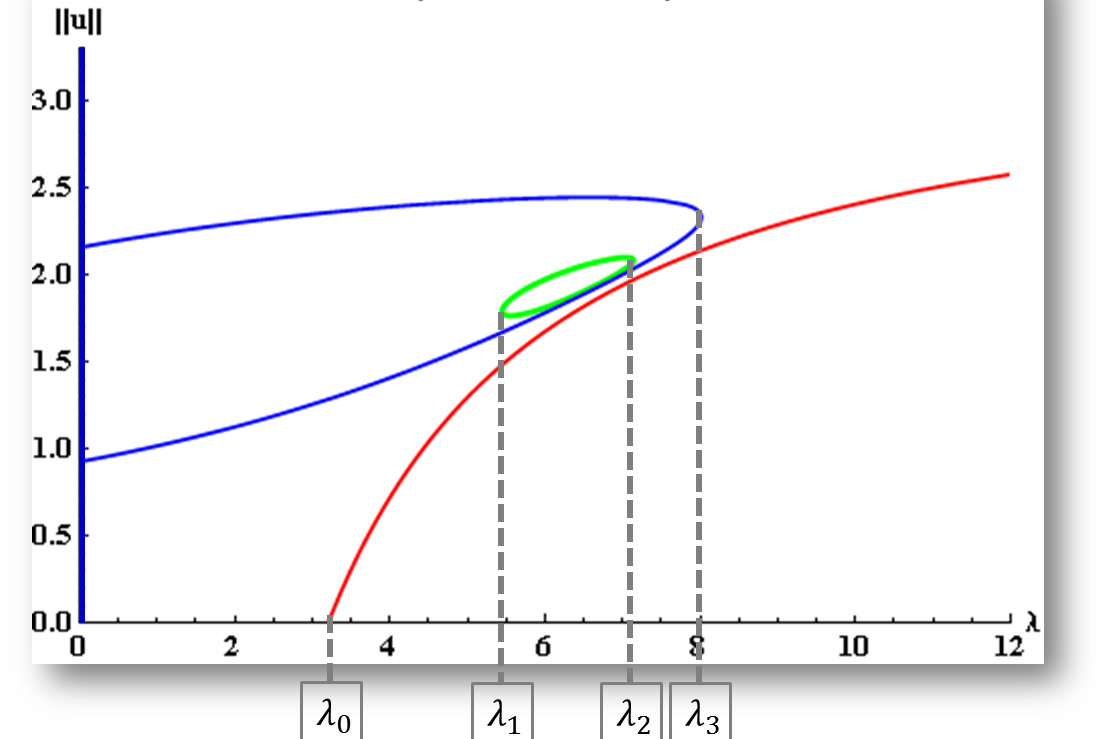# Overview:

My research is primarily focused on developing, parameterizing, and analyzing the dynamics of population models known as reaction diffusion equations which are built on partial differential equations (PDEs) in order to provide a theoretical understanding of the role/consequences of dispersal of populations. This type of modeling falls within an area known as spatial ecology, which is a subdiscipline of mathematical biology. My three main areas of interest all lie at the intersection of dispersal of populations: 1) developing and mathematically analyzing population models to explore effects of conditional dispersal, 2) fitting reaction diffusion models to actual field data to model dispersal abilities of particular organisms, and 3) studying the structure and stability properties of the positive steady state solutions of reaction diffusion equations with nonlinear boundary conditions.

## Research Gallery:### Experimental & Mathematical Study of Density Dependent Dispersal

This collaborative NSF-funded project involves an ecologist at Louisiana State University and a mathematician at University of North Carolina at Greensboro and explores the effects of habitat fragmentation, conditional dispersal, and interspecific interactions on population dynamics from the patch to the landscape level.### Modeling Arthropods of Medical Importance

This project is a collaboration with entomologists at Mississippi State University which applies mathematical modeling to better understand dispersal of medically important arthopods such as bed bugs and ticks.### Mathematical Analysis of Positone/Semipositone Models

An ongoing project that explores the dynamics of reaction diffusion equations arising from models in population dynamics and combustion theory. The main emphasis in this project is the development of new methods in nonlinear analysis to address complexities in the differential equations. Semipostione and positone reaction terms are both included.